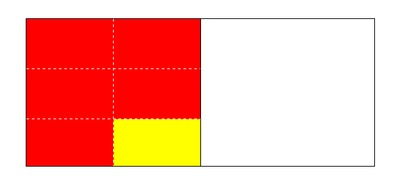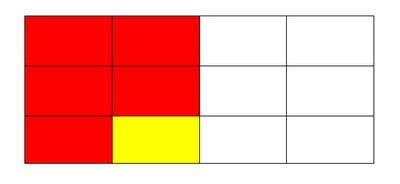# Painting a Wall

Alignments to Content Standards: 5.NF.B

Nicolas is helping to paint a wall at a park near his house as part of a community service project. He had painted half of the wall yellow when the park director walked by and said,

This wall is supposed to be painted red.

Nicolas immediately started painting over the yellow portion of the wall. By the end of the day, he had repainted $\frac56$ of the yellow portion red.

What fraction of the entire wall is painted red at the end of the day?

## IM Commentary

The purpose of this task is for students to find the answer to a question in context that can be represented by fraction multiplication. This task is appropriate for either instruction or assessment depending on how it is used and where students are in their understanding of fraction multiplication. If used in instruction, it can provide a lead-in to the meaning of fraction multiplication. If used for assessment, it can help teachers see whether students readily see that this is can be solved by multiplying $\frac56\times \frac12$ or not, which can help diagnose their comfort level with the meaning of fraction multiplication.

The teacher might need to emphasize that the task is asking for what portion of the total wall is red, it is not asking what portion of the yellow has been repainted.

## Solutions

Solution: Solution 1

In order to see what fraction of the wall is red we need to find out what $\frac56$ of $\frac12$ is. To do this we can multiply the fractions together like so:

$\frac56 \times \frac12 = \frac{5 \times 1}{6 \times 2} = \frac{5}{12}$

So we can see that $\frac{5}{12}$ of the wall is red.

Solution: Solution 2

The solution can also be represented with pictures. Here we see the wall right before the park director walks by:And now we can break up the yellow portion into 6 equally sized parts:Now we can show what the wall looked like at the end of the day by shading 5 out of those 6 parts red.And finally, we can see that if we had broken up the wall into 12 equally sized pieces from the beginning, that finding the fraction of the wall that is red would be just a matter of counting the number of red pieces and comparing them to the total.And so, since 5 pieces of the total 12 are red, we can see that $\frac{5}{12}$ of the wall is red at the end of the day.